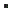How to use calculation fields?This topic explains how to use calculation fields in the SDK.
Calculation fields are defined in the parameters of a module and are added dynamically to the file during runtime. Therefore, they cannot be consulted using the properties of an interface.
Instead they must be approached by the GetFieldVal method of the interface, provided with the field identifier as a parameter. You can retrieve this field identifier with the method GetFieldID based on the code of the calculation field, that can be found in the module parameters.

The samples below use the Accnt interface and are based on the assumption that a numerical calculation field is defined with code 'myBalance'.GetFieldValGetFieldIDC++ double lfBalance = pAccnt->GetFieldVal (pAccnt->GetFieldID ("myBalance")).dblVal;C# double lfBalance = (double)oAccnt.GetFieldVal(oAccnt.GetFieldID("myBalance"));VBS Dim lfBalance lfBalance = oAccnt.GetFieldVal(oAccnt.GetFieldID("myBalance"))VB.NET Dim lfBalance As double lfBalance = oAccnt.GetFieldVal(oAccnt.GetFieldID("myBalance"))